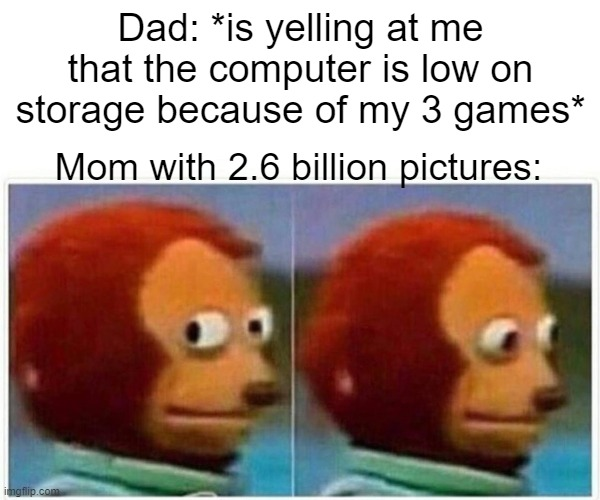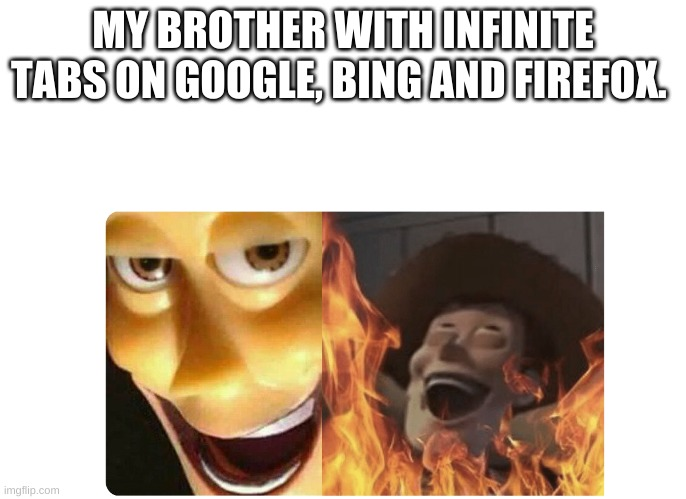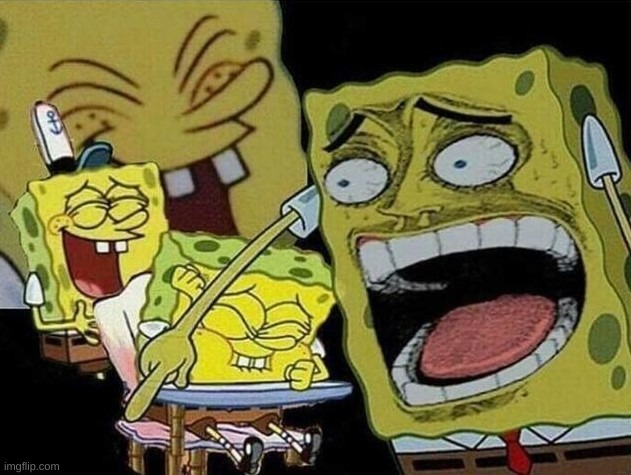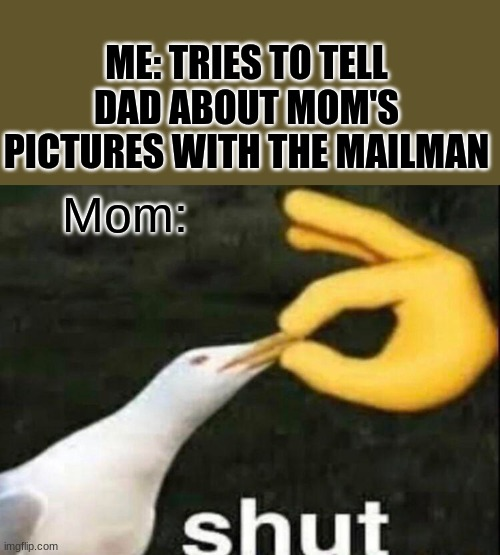# Computer storage spaceshareCaption this Meme
Post Comment
Best first
5 ups, 1m,3 ups, 1m,His cousin with 15 Terabytes of P*rn
0 ups, 1m
thats me bruh
4 ups, 1m,
A picture may be worth a thousand words, but a game is worth a thousand pictures.
1 up, 1m
WarThunder on a 999 GB is only worth about 99999999999999999999999999999999999999999999999999999999999999999999999 pictures
3 ups, 1m2 ups, 1m
Relatable
1 up, 1m
SOOOOO TRUE
1 up, 1m,
my dad has 10^80 x 9 photos
0 ups, 3w
Mom has 69^69^69^69^69^69^69^69^69^69^69^69^69^69^69^69^69^69^69^69^69^69^69^69^69^69^69^69^69^69^69^69^69^69^69^69^69^69^69^69^69^69^69^69^69^69^69^69^69^69^69^69^69^69^69^69^69^69^69^69^69^69^69^69^69^69^69^69^69 photos
1 up, 1m
THIS IS SOOOO RELATABLE!!!
1 up, 1m
lol
0 ups, 1m
Same except my mother has a Google pixels
0 ups, 3w
0 ups, 1m
This is the most relatable thing i have ever seen.....
0 ups, 1m
Can we get a Wubbzy meme on hot
0 ups, 1m
0 ups, 1m,
Most of these pictures would be of your ugly or handsome or beautiful face.Caption this Meme